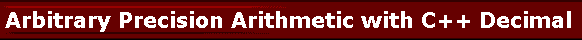# Using Arbitrary Precision Arithmeticwith Math Parser

Some scientific applications or financial applications require the use of a decimal type that can guarantee certain precision when computing mathematical expressions. In C++ it is possible to implement such as type using templates and operator overloading. The user will input an expression that contains decimal numbers and this user supplied formula will need to be parsed and evaluated by the mathematical expression parser in your software.

Bestcode Math Parser for C++ comes with support and usage examples for the MAPM  C++ library . You can plug in the MAPM C++ type to your parser type declaration and set the preprocessor directive _DECIMAL for it all to work.

 #include #include //If you are going to use the parser with a arbitrary precision decimal type such as MAPM then define this: #define _DECIMAL #include "MathParser.h" using namespace std; typedef CMathParser MathParser; //stream output operator for MAPM std::ostream &operator<< (std::ostream &out, MAPM const &mapm){         char a;         mapm.toString(a, 15);         out << a;         return out; } int main(char **args){         cout << "Math Parser Example Expressions" << endl;         try{                 MathParser p;                 cout << "-------------------------------------------------------" << endl;                 //If you compute this using double precision, you are not going to get                 //the correct result.                 //This is (a-b)*(a-b) which is a^2-2*a*b+b^2.                 //But we know that a-b is -1. Therefore, the result is 1. But a double                 //precision number cannot compute a^2-2*a*b+b^2 in this case.                 //But using MAPM, our math parser can do it.                 p.SetExpression("9876543210^2-2*9876543210*9876543211+9876543211^2");                 cout << p.GetExpression() << endl;                 MAPM val = p.GetValue();                 cout << val << endl;                                 cout << "-------------------------------------------------------" << endl;                 cout << "Done.";         }catch(MathParser::ParserException &ex ){                 cout << ex.GetMessage() << endl;                 cout << "Invalid portion of expression is <" << ex.GetInvalidPortionOfExpression() << ">." << endl;         }catch(...){                 cout << "Unexpected error in math parser." << endl;         }         char c;         cin >> c; }

When executed, the output of this example is:

Math Parser Example Expressions
-------------------------------------------------------
9876543210^2-2*9876543210*9876543211+9876543211^2
1.000000000000000E+0
-------------------------------------------------------
Done.

## Limitations of double precision arithmetic

There are limitations to what can be computed correctly using double precision. Rounding errors or overflows cause computations to go wrong. For example:

9876543210^2-2*9876543210*9876543211+9876543211^2

If you use double precision, this expression will produce a wrong answer that depends on the floating point processor. To get the right answer, you need to use an arbitrary precision library such as MAPM as shown above. (Many thanks to Michael C. Ring).

The correct result of this expression is 1. Here we have two values:

a: 9876543210
b:
9876543211

And the expression above is actually the same as:

(a-b)*(a-b)

Since a-b is -1, the value of the above expression is 1.

Some applications, typically scientific and financial applications, need to work with very large numbers, or they need to work with arbitrary precision where rounding errors or numeric overflows cause incorrect results. C++ templates and operator overloading features make it possible to write pluggable types that can be used instead of the built in double precision type. MAPM is one such library and Math Parser for C++ provides support with it. Math Parser for C++ also ships a copy of this library for your convenience.

webmaster@gobestcode.com